JEE  >  Test: Single Correct MCQs: Simple Harmonic Motion (Oscillations) | JEE Advanced

# Test: Single Correct MCQs: Simple Harmonic Motion (Oscillations) | JEE Advanced

Test Description

## 11 Questions MCQ Test | Test: Single Correct MCQs: Simple Harmonic Motion (Oscillations) | JEE Advanced

Test: Single Correct MCQs: Simple Harmonic Motion (Oscillations) | JEE Advanced for JEE 2023 is part of JEE preparation. The Test: Single Correct MCQs: Simple Harmonic Motion (Oscillations) | JEE Advanced questions and answers have been prepared according to the JEE exam syllabus.The Test: Single Correct MCQs: Simple Harmonic Motion (Oscillations) | JEE Advanced MCQs are made for JEE 2023 Exam. Find important definitions, questions, notes, meanings, examples, exercises, MCQs and online tests for Test: Single Correct MCQs: Simple Harmonic Motion (Oscillations) | JEE Advanced below.
Solutions of Test: Single Correct MCQs: Simple Harmonic Motion (Oscillations) | JEE Advanced questions in English are available as part of our course for JEE & Test: Single Correct MCQs: Simple Harmonic Motion (Oscillations) | JEE Advanced solutions in Hindi for JEE course. Download more important topics, notes, lectures and mock test series for JEE Exam by signing up for free. Attempt Test: Single Correct MCQs: Simple Harmonic Motion (Oscillations) | JEE Advanced | 11 questions in 20 minutes | Mock test for JEE preparation | Free important questions MCQ to study for JEE Exam | Download free PDF with solutions
 1 Crore+ students have signed up on EduRev. Have you?
Test: Single Correct MCQs: Simple Harmonic Motion (Oscillations) | JEE Advanced - Question 1

### Two bodies M and N of equal masses are suspended from two separate massless springs of spring constants k1 and k2 respectively. If the two bodies oscillate vertically such that their maximum velocities are equal, the ratio of the amplitude of vibration of M to that of N is

Detailed Solution for Test: Single Correct MCQs: Simple Harmonic Motion (Oscillations) | JEE Advanced - Question 1

Both the bodies oscillate in simple harmonic motion, for which the maximum velocities will be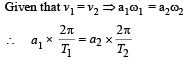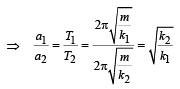Test: Single Correct MCQs: Simple Harmonic Motion (Oscillations) | JEE Advanced - Question 2

### A particle free to move along the x-axis has potential energy given by U(x) = k [1–exp(–x2)] for -∞ < x < + ∞, where k is a positive constant of appropriate dimensions. Then

Detailed Solution for Test: Single Correct MCQs: Simple Harmonic Motion (Oscillations) | JEE Advanced - Question 2

Let us plot the graph of the mathematical equation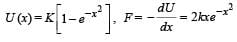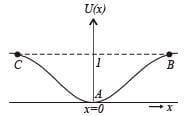From the graph it is clear that the potential energy is minimum at x =  0. Therefore, x = 0 is the state of stable equilibrium. Now if we displace the particle from x = 0 then for displacements the particle tends to regain the position x = 0 with a force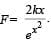Therefore for small
values of x we have F ∝ x.

Test: Single Correct MCQs: Simple Harmonic Motion (Oscillations) | JEE Advanced - Question 3

### The period of oscillation of a simple pendulum of length L suspended from the roof of a vehicle which moves without friction down an inclined plane of inclination α , is given by

Detailed Solution for Test: Single Correct MCQs: Simple Harmonic Motion (Oscillations) | JEE Advanced - Question 3

As shown in the figure, gsina is the pseudo acceleration applied by the observer in the accelerated frame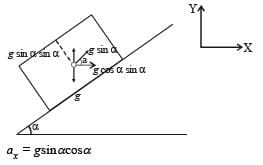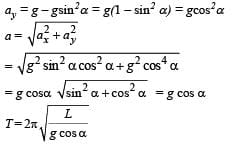NOTE : Whenever point of suspension is accelerating
use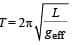Test: Single Correct MCQs: Simple Harmonic Motion (Oscillations) | JEE Advanced - Question 4

A particle executes simple harmonic motion between x = -A and x = +A. The time taken for it to go from 0 to A/2 is T1 and to go from A/2 to A is T2. Then

Detailed Solution for Test: Single Correct MCQs: Simple Harmonic Motion (Oscillations) | JEE Advanced - Question 4

The velocity of a body executing S.H.M. is maximum at its centre and decreases as the body proceeds to the extremes. Therefore if the time taken for the body to go from O to A/2 is T1 and to go from A/2 to A is T2 then obviously T1 < T2

Test: Single Correct MCQs: Simple Harmonic Motion (Oscillations) | JEE Advanced - Question 5

For a particle executing SHM the displacement x is given by x = A coswt. Identify the graph which represents the variation of potential energy (PE) as a function of time t and displacement xDetailed Solution for Test: Single Correct MCQs: Simple Harmonic Motion (Oscillations) | JEE Advanced - Question 5

NOTE : In S.H.M., at extreme position, P.E. is maximum when
t = 0, x = A.
i.e., at time t = 0, the particle executing S.H.M. is at its extreme position.
Therefore P.E. is max. The graph I and III represent the above characteristics.

Test: Single Correct MCQs: Simple Harmonic Motion (Oscillations) | JEE Advanced - Question 6

A simple pendulum has time period T1. The point of suspension is now moved upward according to the relation y = Kt2, (K = 1 m/s2) where y is the vertical displacement. The time period now becomes T2. The ratio of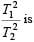(g = 10 m/s2)

Detailed Solution for Test: Single Correct MCQs: Simple Harmonic Motion (Oscillations) | JEE Advanced - Question 6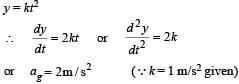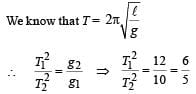[∵g1 = 10 m/s2 and g2 = g + 2 = 12 m/s2]

Test: Single Correct MCQs: Simple Harmonic Motion (Oscillations) | JEE Advanced - Question 7

The x-t graph of a particle undergoing simple harmonic motion is shown below. The acceleration of the particle at t = 4 / 3 s is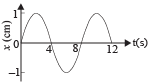Detailed Solution for Test: Single Correct MCQs: Simple Harmonic Motion (Oscillations) | JEE Advanced - Question 7

From the graph it is clear that the amplitude is 1 cm and the time period is 8 second. Therefore the equation for the S.H.M. is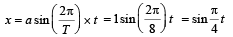The velocity (v) of the particle at any instant of time ‘t’ is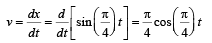The acceleration of the particle is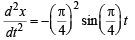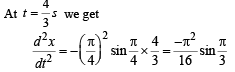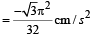Test: Single Correct MCQs: Simple Harmonic Motion (Oscillations) | JEE Advanced - Question 8

A uniform rod of length L  and mass M is pivoted at the centre. Its two ends are attached to two springs of equal spring constants k. The springs are fixed to rigid supports as shown in the figure, and the rod is free to oscillate in the horizontal plane.
The rod is gently pushed through a small angle θ in one direction and released. The frequency of oscillation is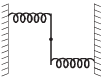Detailed Solution for Test: Single Correct MCQs: Simple Harmonic Motion (Oscillations) | JEE Advanced - Question 8

Figure shows the rod at an angle θ with respect to its equilibrium position. Both the springs are stretched by length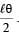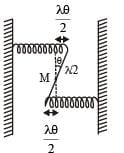The restoring torque due to the springs τ = – 2 (Restoring force) × perpendicular distance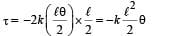... (i)
If I is the moment of inertia of the rod about M then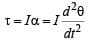… (ii)

From (i) & (ii) we get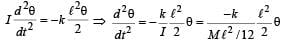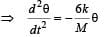Comparing it with the standard equation of rotational SHM we get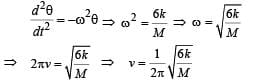Test: Single Correct MCQs: Simple Harmonic Motion (Oscillations) | JEE Advanced - Question 9

The mass M shown in the figure oscillates in simple harmonic motion with amplitude A. The amplitude of the point P is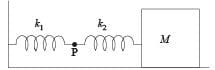Detailed Solution for Test: Single Correct MCQs: Simple Harmonic Motion (Oscillations) | JEE Advanced - Question 9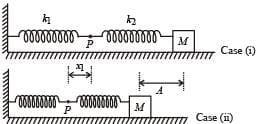In case (ii), the springs are shown in the maximum compressed position. If the spring of spring constant k1 is compressed by x1 and that of spring constant k2 is compressed by x2 then

x1 + x= A … (i)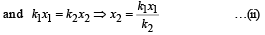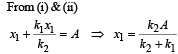Test: Single Correct MCQs: Simple Harmonic Motion (Oscillations) | JEE Advanced - Question 10

A point mass is subjected to two simultaneous sinusoidal displacements in x-direction, x1(t) = A sin wt and x2(t) =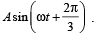Adding a third sinusoidal displacement x3(t) = B sin (ωt + φ) brings the mass to a complete rest. The values of B and φ are

Detailed Solution for Test: Single Correct MCQs: Simple Harmonic Motion (Oscillations) | JEE Advanced - Question 10

Two sinusoidal displacements have amplitude A each, with a phase difference of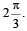It is given that sinusoidal displacement x3(t) brings the mass to a complete rest. This is possible when the amplitude of third is A and is having a phase difference of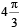with
respect to x1 (t) as shown in the figure.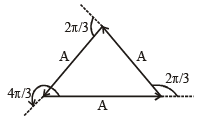Test: Single Correct MCQs: Simple Harmonic Motion (Oscillations) | JEE Advanced - Question 11

A small block is connected to one end of a massless spring of un-stretched length 4.9 m. The other end of the spring (see the figure) is fixed. The system lies on a horizontal frictionless surface. The block is stretched by 0.2 m and released from rest at t = 0. It then executes simple harmonic motion with angular frequency ω = π/3 rad/s. Simultaneously at t = 0, a small pebble is projected with speed v form point P at an angle of 45° as shown in the figure. Point P is at a horizontal distance of 10 m from O. If the pebble hits the block at t = 1 s, the value of v is (take g = 10 m/s2)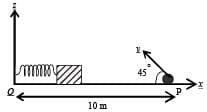Detailed Solution for Test: Single Correct MCQs: Simple Harmonic Motion (Oscillations) | JEE Advanced - Question 11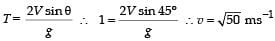Use Code STAYHOME200 and get INR 200 additional OFF Use Coupon Code
Information about Test: Single Correct MCQs: Simple Harmonic Motion (Oscillations) | JEE Advanced Page
In this test you can find the Exam questions for Test: Single Correct MCQs: Simple Harmonic Motion (Oscillations) | JEE Advanced solved & explained in the simplest way possible. Besides giving Questions and answers for Test: Single Correct MCQs: Simple Harmonic Motion (Oscillations) | JEE Advanced, EduRev gives you an ample number of Online tests for practice